Pure and Applied Mathematics Journal
Volume 4, Issue 3, June 2015, Pages: 66-69

Difficulties and Errors of 1st Grade Computer Teaching and Technology Department Students on Discontinuity Types of Partial Functions and Some Certain Functions

Melis Minisker

Mustafa Kemal University, Department of Mathematics, Antakya, Hatay, Turkey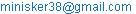Melis Minisker. Difficulties and Errors of 1st Grade Computer Teaching and Technology Department Students on Discontinuity Types of Partial Functions and Some Certain Functions. Pure and Applied Mathematics Journal. Vol. 4, No. 3, 2015, pp. 66-69. doi: 10.11648/j.pamj.20150403.11

Abstract: The aim of this study is to investigate difficulties and errors of 1st grade Computer Teaching and Technology Department students on discontinuity kinds of partial functions and some certain functions. The data is gathered by the questionnaire containing 10 open ended questions. The answers of students are categorized according to 0-3 points’ schema. Based on the results and detailed analysis of students’ responses it is observed that students have many difficulties on finding the right and left hand side limit of partial functions on specific points. So it leads errors on determining the discontinuity types of these functions. Moreover, the results of analysis show that students have some difficulties on determining the discontinuity of some specific functions.

Contents

1. Introduction

The studies of new teaching activities and learning models have been searched for long years. These studies are going on and are increasing nowadays. The effect of new activities on teaching is also widely researched in teaching Mathematics. For example Ucecam and Minisker (2014) considered the effect of guidance activities on40 students studying in 8th branch, at 2012 - 2013 academic year in a special primary school classroom in the district of Hatay Dortyol. This research is designed as pretest / posttest experiment and control group quasi-experimental design model. The experimental group consists of first half of these students and the control group consists of the other half.

Total of 12 guidance activities program has been implemented to experimental group twice a week for 6 weeks. Each activity is applied at the end of the courses for 60 minutes. There has not been any action applied to control group during this time. The mathematics achievement test (MAT) and scale of attitudes towards mathematics (SATM) is applied to all groups as the data collection tool in research.

At the end of the research; a significant difference about mathematics success are found in favor of the experimental group students, between 8th branch students who participated in the experimental group applied guidance activities and the students who did not receive guidance activities in the control group. At the same time; a significant difference about attitudes towards mathematics are found in favor of the experimental group students, between 8th branch students who participated in the experimental group guidance activities and the students who did not receive guidance activities in the control group.

Another study is prepared by Keskin Deniz and Minisker (2014) which aimed to determine effect of reading comprehension skill on the academic achievement of fifth grade students of elementary schools in math lesson. The factors including gender, habit of reading books, socio-economic level, and having difficulty while solving problems were also studied using correlational comparative survey model.

The population of the study includes the 5th grade students from 85 public elementary schools located in the city of Sahinbey of the Gaziantep province in the spring semester of the 2011 – 2012 academic year. The sample includes 362 students from the schools with different socio-economical areas by selection using "purposively sampling" method.

Reading Comprehension Test and Mathematics Achievement Test were used to collect data for the investigation. First of all, Reading Comprehension Test consisting of 28 multiple choice questions was administered to the participants. Secondly, Math Problem Test including 22 questions was applied to the participants.

SPSS software programme (version 15.0) was used to analyse the data. Frequency (f), Percentage (%), t-test (t), the One-Way Analysis of Variance (ANOVA) and Pearson product-moment correlation coefficients were used in order to investigate the relationship between the skill of "reading comprehension" and "achievement in math lesson".

Limit and continuity concepts have been widely searched for years. As Cornu(1991) tells, limit is one of the most basic concepts of analysis because of its relationship between the concepts continuity, derivative and approximation theory. Past studies about limit and continuity concepts indicate that students have conceptual errors about limit and continuity and they have difficulties in learning these concepts. (Davis &Vinner, 1986; Tall & Vinner, 1981; Cornu, 1991; Williams, 1991; Szydlik, 2000).

Generally students think about limit concept just as a maximum value to reach and it means a boundary value like the limit of credit cards. Williams(1991) and Jordan(2005) indicate in their studies that the daily use of limit given above can be admitted similar to limit of functions by students. Past studies show that students make conceptual errors when they find a relationship between a function’s limit and a function’s domain.(Bergthold, 1999; Jordaan, 2005; Akbulut & Işık, 2005).

On the other hand studies indicate that students have conceptual errors that limit is a value that can never be reached. (Williams, 1989, 1991; Szydlik, 2000; Akbulut & Işık, 2005).

Tall and Vinner (1981) tells that the continuity concept is constructed upon informal definitions instead of formal definitions. In daily use the word ‘continuity’ is admitted ‘without distances’. As a student understands continuity concept based upon this definition, he makes conceptual errors when understanding this concept as a mathematical concept and meets difficulties. Students think that a graph of a continous function does not contain any breaks and distances.

In this study we find the difficulties of computer teaching and tecnology department students in 1st class upon determining the continuity of a given function and determining the discontinuity points and discontinuity types. The difference of this study from past studies about conceptual errors in limit and continuity is that we scan the difficulties of students on determining discontinuity types of functions on the points where the function is discontinous.

2. Conceptual Model and Method

Participants are 36 students of 1st grade in Computer Teaching and Technology Department in Mustafa Kemal University in Faculty of Education.

In order to determine the difficulties of students on determining discontinuity types of certain functions, a questionnaire which consists of 10 open-ended questions is prepared. In the questionnaire 4 questions contain partial functions, 4 questions contain rational functions and the remaining 2 questions contain different types of functions such that absolute value function, signum function e.t.c. The questionnaire is given in Appendix A.

The aim of this study is to determine the difficulties of 1st grade Computer Teaching and Technology Department Students on determining the discontinuity types of certain fuctions. A questionnaire which consists of 10 open-ended questions is pepared and evaluated to 36 students. The answers of students are reviewed. The points are scored 0, 1, 2 and 3. The questions which are scored 1 or 2 are scanned carefully and the difficulties of students on continous functions are determined.

The evaluation criterion is given in Table 1 below.

Table 1. Evaluation Criterion and Points.

 Point Criterion 0 The question is not answered or the answer is wrong 1 The answer is almost correct. 2 The way of solution is correct, but there are operation mistakes. 3 The way of solution and the answer is correct.

The frequency and percentage rates of correct answers and arithmetic means are given in Table 2.

Table 2. The frequency and percentage rates of correct answers and arithmetic means.

 CRITERION 0 1 2 3 QUESTION n % n % n % n %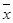1 20 56 4 11 2 6 10 28 1,05 2 10 28 5 14 5 14 16 45 2,1 3 30 83 2 6 4 11 0 0 0,27 4 8 22 1 3 3 8 24 67 2,19 5 17 47 5 14 9 25 4 11 0,97 6 22 61 9 25 2 6 3 8 0,61 7 12 33 4 11 3 8 16 45 1,61 8 11 31 0 0 10 28 14 39 1,72 9 15 42 10 28 10 28 1 3 0,91 10 16 45 1 3 6 17 3 8 0,61

If Table 2 is scanned carefully the percentage of success is the highest in the 4th question (67%). In the 2nd, 7nd and 8th questions the percentage of success decrease (45%, 45%, 39%). In the 1st question the percentage of success gets lower(28%). In the 5th, 6th, 9th and 10th questions the percentage of success is too low (11%, 8%, 3%, 8%).

General mistakes and conceptual errors are divided into 4 categories:

(1)       Making certain mistakes in taking left hand limit or right hand limit in partial functions

(2)       Forgetting to take left or right hand limit or ignoring right and left hand limits and taking limit directly

(3)       Mistakes in determining discontinuity types

(4)       Discontinuity is scanned in wrong points

In the 2nd, 4th, 6th, 7th and 8th questions students made certain mistakes in taking left hand limit or right hand limit. 69% of students have made similar mistakes in these questions. 25% of students have made operation error in the 8th question. For example in the 7th question Student 1’s answer is given below:

Student 1:and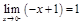So the function is continous at x=0.

In the 9th and 5th questions 17% of students forgot to take left or right hand limit or ignored right hand and left hand limits and took limit directly. 67% of the students who made similar mistakes have for gotton to take left hand limit in the 9th question. For example Student 2’s answer is given below:

Student 2: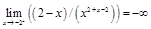So the function is discontinous at x=-2.

It can be seen that the left hand limit is ignored.

In the 9th, 7th, 6th, 5th, 3rd and 1st questions 58% of students made mistakes in determining discontinuity types. 14% of these students who made similar mistakes have pointed out that there exists removable discontinuity at x=-2 in the 9th question. 10% of the students who made similar types of mistakes have pointed out that there exists jump discontinuity at x=-2 in the 9th question. 19% of the students who made similar types of mistakes have pointed out that there exists removable discontinuity at x=-1 in the 6th question. As an example in the 9th question Student 3 has given the answer below:

Student 3.Since f(x)= ((|x|+2)/(x²+x-2))and the function is undefined at x=-2 and x=1. So there exists removable discontinuity at these points.

36% of students determined discontinuity in wrong points in the 1st, 3rd, 6th, 2nd and 7th questions. 38% of students who made these mistakes have determined discontinuity at x=1 instead of x=0 in the 1st question. 31% of these students have determined discontinuity at x=0 instead of x=1 in the 3rd question. For example in the 1st question Student 4 has given the answer below:

Student 4: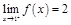and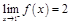and so the function has jump discontinuity at x=1.

But the discontinuity must be scanned at the point x=0 instead of x=1. Because f(x)=x+|x|.

As a result it can be observed that the most frequently occuring mistakes are taking left hand limit or right hand limit wrong in partial functions (69%). These mistakes occur in the 2nd, 4th, 6th, 7th and 8th questions. 58% of students made mistakes in determining discontinuity types in the 9th, 7th, 6th, 5th, 3rd and 1st questions. 36% of students determined discontinuity in wrong points in the 1st, 3rd, 6th, 2nd and 7th questions. 17% of students forgot to take left or right hand limit or ignored right hand and left hand limits and took limit directly. These calculations lead us to the fact that the conceptual errors in determining discontinuity of functions are based upon conceptual errors in taking limit and determining discontinuity types on critical points.

3. Conclusion

These calculations lead us to the fact that the conceptual errors in determining discontinuity of functions are based upon conceptual errors in taking limit and determining discontinuity types on critical points. These findings support the findings of Tall and Vinner (1981) about conceptual errors on continuity of functions. Basturk and Donmez (2011) evaluated a questionnaire to 37 teacher candidates studying in Secondary School Mathematics Education to investigate student teachers’ misconceptions related to the limit and continuity concept. They obtained that the student teachers have misconceptions about mixing limit and continuity concepts. For example, if a function has a limit at one point it should be defined and continous at that point. Barak (2007) showed that students have misconceptions about epsilon-delta definition, definition of limit concept, a function’s limit at a point, limits on the right and the left, relation between limit and continuity concepts. These findings coincide with our findings in this study.

Appendix A

Questionnaire

1.         The function f:R®R f(x)=x+is given. Are there any points where the function is discontinous? If there are such points determine the discontinuity types.

2.         f(x)=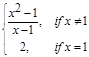is given. Are there any points where the function is discontinous? If there are such points determine the discontinuity types.

3.         f(x)=x2+Sgn(x2-1) is given. Are there any points where the function is discontinous? If there are such points determine the discontinuity types.

4.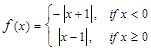is given. Are there any points where the function is discontinous? If there are such points determine the discontinuity types.

5.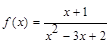is given. Are there any points where the function is discontinous? If there are such points determine the discontinuity types.

6.         f(x)=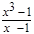is given. Are there any points where the function is discontinous? If there are such points determine the discontinuity types.

7.         f(x)=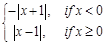is given. Are there any

8.         points where the function is discontinous? If there are such points determine the discontinuity types.

9.         f(x)=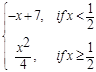is given. Are there anypoints where the function is discontinous? If there are such points determine the discontinuity types.

10.      f(x):R\{-2, 1}® Rf(x)=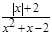is given. Are there any points where the function is discontinous? If there are such points determine the discontinuity types.

11.      f(x):R\{ 1}®Rf(x)=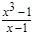is given. Are there any points where the function is discontinous? If there are such points determine the discontinuity types.

References

1. K. Akbulut and A. Işık, "Examining interactive teaching method to understand limit concept and misconceptions in this period", Kastamonu Education Journal, Vol13 (2),pp. 497-512, 2005.
2. B. Barak, "Determining Misconceptions in Limit Concept", MSC Thesis, Balıkesir University, Balıkesir, 2007.
3. S. Basturk and G. Dönmez, "Mathematics Student Teachers’ Misconceptions on the Limit and Continuity Concepts", Necatibey Faculty of Education Electronic Journal of Science and Mathematics Education, Vol 5 (1), pp.225-249, 2011.
4. T.A. Bergthold, Patterns of analytical thinking and knowledge use in students’ early understanding of the limit concept,Unpublished Doctoral Dissertation, University of Oklahama,Oklahama,1999.
5. B. Cornu, Limits. In D. Tall (Eds.), Advanced mathematical thinking, Dordrect, The Netherlands: Kluwer Academic, pp.153-166, 1991.
6. R.B. Davis and S. Vinner, The notion of limit; some seemingly an avoidable misconception stages, J. Math. Behav., Vol. 5, pp.281–303, 1986.
7. T. Jordaan, Misconceptions of the limit concept in a mathematics course for engineering students, Unpublished Master of Science Dissertation, University of South Africa, 2005.
8. J.E. Szydlik, "Mathematical beliefs and conceptual understanding of the limit of a function", Journal for Research in Mathematics Education, Vol 31(3), pp.258-276, 2000.
9. D.Tall and S. Vinner, "Concept image and concept definition in mathematics with particular reference to limits and continuity",Educational Studies in Mathematics, Vol. 12, pp.151–169, 1981.
10. K. Ucecam and M. Minisker, "8thGrade Students’ Attitudes Towards Mathematics Applied Guidance Activities and Mathematics Course Success Applied Guidance Activities", MSC Thesis, Mustafa Kemal University, Hatay, Turkey,2014.
11. M. KeskinDeniz and M. Minisker, "Studying the Relationship Between Reading Comprehension Skill and Academic Achivement of The Elementary School 5th Grade Students in Math Lesson", MSC Thesis, Mustafa Kemal University, Hatay, Turkey,2014.

Article ToolsAbstractPDF(180K)